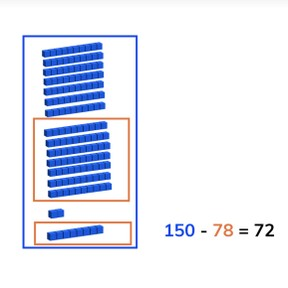Subtraction to 1,000 crossing tens and hundreds

# Subtraction to 1,000 crossing tens and hundreds

Subtract numbers to 1,000 crossing tens and hundreds

No account needed.8,000 schools use Gynzy92,000 teachers use Gynzy1,600,000 students use Gynzy

## General

Students learn to subtract numbers to 1,000 crossing tens and hundreds.

## Standards

CCSS.Math.Content.2.NBT.B.7

## Learning objective

Students will be able to subtract numbers to 1,000 crossing tens and hundreds.

## Introduction

Show students the three pretzels in parts. Ask students if these math problems are correct. As they are not correct, as students to drag the pretzel parts around to form correct math problems. Then ask them to talk through the steps of solving the problems out loud.

## Instruction

Explain that you can subtract by counting. You first count how many there are in total, this is the first number of the subtraction problem (the minuend). Then you count how many are taken away, which is the second number of the subtraction problem (the subtrahend). When you take the second from the first you are left with the difference. Show this on the interactive whiteboard using the blocks, and discuss the different problems. This includes problems in which the second number (the subtrahend) has hundreds, tens, and ones. Check that students are able to solve a few problems on their own or in pairs with the given problems with blocks.Ask them to say the problem they are solving out loud, and to say the steps they are taking out loud. Next explain that you can solve these subtraction problems in different ways. Show that you can use a number line to subtract. You can also split the subtrahend and take away the hundreds, tens, and ones in steps. You can also choose to take away the subtrahend in one step. Emphasize to students that they should first carefully look at the problem and then decide which method to use, because all of them work, but some are easier to use on different math problems. Ask students to solve a few problems individually or in pairs, explaining their strategy. Finally walk through the steps of a story problem with the class. Explain that you first determine what kind of math problem it is, then you determine which numbers are important for the problem. You say or write the problem and finally solve the problem. Do one story problem as a class and then ask students to solve another individually or in pairs.

Check that students are able to subtract numbers to 1,000 crossing tens and hundreds by asking the following questions:
- What do you look at when you want to solve a story problem?
- How do you solve 463 - 185?

## Quiz

Students are given problems in which they must solve using visual support, solve an abstract problem, and story problems. Encourage students to explain their strategy and walk through the steps of the subtraction problems.

## Closing

Discuss with students that it is important to be able to subtract numbers to 1,000 crossing tens and hundreds so you can determine how many you have left. Ask students to solve the problems on the interactive whiteboard. Have them explain their strategy. Close the lesson with a game. Have students come up to the board one by one to drag a pumpkin away. If they find a ghost, they're out and have to sit down. If they find a number they can stay. When both numbers have been found, solve the problem as a class.

## Teaching tips

Students who have difficulty with subtraction can make use of the number line to support their subtraction. Remind students that they can take different sized steps on the number line.

## Instruction materials

MAB blocks or manipulatives, number line

### The online teaching platform for interactive whiteboards and displays in schools

• Save time building lessons

• Manage the classroom more efficiently

• Increase student engagement